The GLIMMIX Procedure

Quadrature methods, like the Laplace approximation, approximate integrals. If you choose METHOD=QUAD for a generalized linear mixed model, the GLIMMIX procedure approximates the marginal log likelihood with an adaptive Gauss-Hermite quadrature rule. Gaussian quadrature is particularly well suited to numerically evaluate integrals against probability measures (Lange 1999, Ch. 16). And Gauss-Hermite quadrature is appropriate when the density has kernel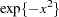and integration extends over the real line, as is the case for the normal distribution. Suppose that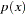is a probability density function and the function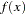is to be integrated against it. Then the quadrature rule is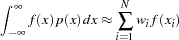where
denotes the number of quadrature points, the
are the quadrature weights, and the
are the abscissas. The Gaussian quadrature chooses abscissas in areas of high density, and ifis continuous, the quadrature rule is exact ifis a polynomial of up to degree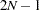. In the generalized linear mixed model the roles ofandare played by the conditional distribution of the data given the random effects, and the random-effects distribution, respectively. Quadrature abscissas and weights are those of the standard Gauss-Hermite quadrature (Golub and Welsch 1969; see also Table 25.10 of Abramowitz and Stegun 1972; Evans 1993).

A numerical integration rule is called adaptive when it uses a variable step size to control the error of the approximation. For example, an adaptive trapezoidal rule uses serial splitting of intervals at midpoints until a desired tolerance is achieved. The quadrature rule in the GLIMMIX procedure is adaptive in the following sense: if you do not specify the number of quadrature points (nodes) with the QPOINTS= suboption of the METHOD=QUAD option, then the number of quadrature points is determined by evaluating the log likelihood at the starting values at a successively larger number of nodes until a tolerance is met (for more details see the text under the heading "Starting Values" in the next section). Furthermore, the GLIMMIX procedure centers and scales the quadrature points by using the empirical Bayes estimates (EBEs) of the random effects and the Hessian (second derivative) matrix from the EBE suboptimization. This centering and scaling improves the likelihood approximation by placing the abscissas according to the density function of the random effects. It is not, however, adaptiveness in the previously stated sense.

#### Objective Function

Let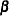denote the vector of fixed-effects parameters and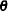the vector of covariance parameters. For quadrature estimation in the GLIMMIX procedure,includes the G-side parameters and a possible scale parameter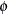, provided that the conditional distribution of the data contains such a scale parameter.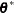is the vector of the G-side parameters. The marginal distribution of the data for subjectin a mixed model can be expressed as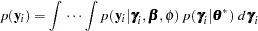Suppose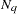denotes the number of quadrature points in each dimension (for each random effect) anddenotes the number of random effects. For each subject, obtain the empirical Bayes estimates of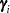as the vector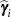that minimizes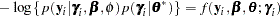If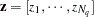are the standard abscissas for Gauss-Hermite quadrature, and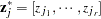is a point on the-dimensional quadrature grid, then the centered and scaled abscissas are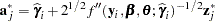As for the Laplace approximation,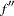is the second derivative matrix with respect to the random effects,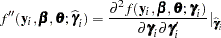These centered and scaled abscissas, along with the Gauss-Hermite quadrature weights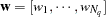, are used to construct the-dimensional integral by a sequence of one-dimensional rules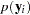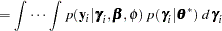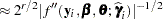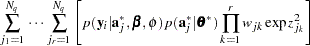The right-hand side of this expression, properly accumulated across subjects, is the objective function for adaptive quadrature estimation in the GLIMMIX procedure.

If you select the quadrature rule with a single quadrature point, namely

```   proc glimmix method=quad(qpoints=1);
```

the results will be identical to METHOD=LAPLACE. Computationally, the two methods are not identical, however. METHOD=LAPLACE can be applied to a considerably larger class of models. For example, crossed random effects, models without subjects, or models with non-nested subjects can be handled with the Laplace approximation but not with quadrature. Furthermore, METHOD=LAPLACE draws on a number of computational simplifications that can increase its efficiency compared to a quadrature algorithm with a single node. For example, the Laplace approximation is possible with unbounded covariance parameter estimates (NOBOUND option in the PROC GLIMMIX statement) and can permit certain types of negative definite or indefinite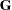matrices. The adaptive quadrature approximation with scaled abscissas typically breaks down whenis not at least positive semidefinite.

As the number of random effects grows—for example, if you have nested random effects—quadrature quickly becomes computationally infeasible, due to the high dimensionality of the integral. To this end it is worthwhile to clarify the issues of dimensionality and computational effort as related to the number of quadrature nodes. Suppose that the A effect has 4 levels and consider the following statements:

```   proc glimmix method=quad(qpoints=5);
class A id;
model y = / dist=negbin;
random A / subject=id;
run;
```

For each subject, computing the marginal log likelihood requires the numerical evaluation of a four-dimensional integral. As part of this evaluation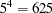conditional log likelihoods need to be computed for each observation on each pass through the data. As the number of quadrature points or the number of random effects increases, this constitutes a sizable computational effort. Suppose, for example, that an additional random effect with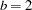levels is added as an interaction. The following statements then require evaluation of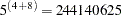conditional log likelihoods for each observation one each pass through the data:

```   proc glimmix method=quad(qpoints=5);
class A B id;
model y = / dist=negbin;
random A A*B / subject=id;
run;
```

As the number of random effects increases, Laplace approximation presents a computationally more expedient alternative.

If you wonder whether METHOD=LAPLACE would present a viable alternative to a model that you can fit with METHOD=QUAD, the "Optimization Information" table can provide some insights. The table contains as its last entry the number of quadrature points determined by PROC GLIMMIX to yield a sufficiently accurate approximation of the log likelihood (at the starting values). In many cases, a single quadrature node is sufficient, in which case the estimates are identical to those of METHOD=LAPLACE.Previous Page | Next Page | Top of Page